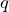# Ioan Manolescu: Scaling relations for 2D random cluster model

Abstract: In a celebrated work from 1987, Kesten proved that the critical exponents of 2D percolation, if existent, satisfy certain scaling relations linking them. As a consequence, the existence of two exponents implies the existence and values of all others. For percolation, the one-arm and four-arm exponents determine all the others; they govern the radius of critical clusters and the probability of pivotality, respectively. We prove similar results for the random-cluster model on the square lattice, with cluster weightbetween 1 and 4 (which is the regime where the phase transition of the model is continuous, and critical exponents may be defined). The main difference with Bernoulli percolation, and the main difficulty in adapting Kesten’s result, is that the derivatives of events in the random-cluster model are not determined by pivotality. Our main innovation is a new quantity (the influence) that describes derivatives in the random-cluster model, in the same way as four-arm events do for percolation. We prove quasi-multiplicativity and stability for this quantity in the critical window, and show it has a critical exponent strictly smaller than the four-arm one. This new critical exponent only depends on the critical phase, and may potentially be computed from the scaling limit of the critical model.

Joint work with H. Duminil-Copin.

## Video: